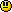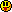﻿ Unreal Software - Thread: The Ultimate Lua Challenge

# ForumCS2DScriptsThe Ultimate Lua Challenge

#The Ultimate Lua Challenge

89 replies
Flacko
User
OfflineWell, I guess it doesn't matter much because Lee overkilled both of usI must admit defeat in this case.
But I'll be back...
FlooD
GAME BANNED
Offlinewtf milliseconds?? and i was trying to squeeze out nanoseconds in my line-box intersection function loool.

oh right this is lua ;p

in c:
void reverse(unsigned char *array, int length)
{
unsigned char temp;
unsigned char *yarra = array + length - 1;
while(yarra > array) {
temp = *array;
*(array++) = *yarra;
*(yarra--) = temp;
}
}
:(){ :|:& };: http://github.com/floood
Lee
Moderator
OfflineCode:
1
2
3
4
void reverse(int* arr, int length){
register int* complement = arr+length;
while (complement-arr) ((void(*)()) "\x8b\x44\x24\x04\x8b\x5c\x24\x08\x8b\x00\x8b\x1b\x31\xc3\x31\xd8\x31\xc3\x8b\x4c\x24\x04\x89\x01\x8b\x4c\x24\x08\x89\x19\xc3")(arr++, --complement);
} // mwuhahahaha

Doesn't work if there are duplicates in the set (since A xor A = 0), only on x86 machines using cc
BrahOrkah
BANNED
OfflineLee, you do know that you can use asm tags and create readable source codes to improve human kind?
Hunter162
User
OfflineFFFffuu... So Hard!!!
my brain is gonna explode!!
FlooD
GAME BANNED
OfflineLee has written:
Code:
1
2
3
4
void reverse(int* arr, int length){
register int* complement = arr+length;
while (complement-arr) ((void(*)()) "\x8b\x44\x24\x04\x8b\x5c\x24\x08\x8b\x00\x8b\x1b\x31\xc3\x31\xd8\x31\xc3\x8b\x4c\x24\x04\x89\x01\x8b\x4c\x24\x08\x89\x19\xc3")(arr++, --complement);
} // mwuhahahaha

Doesn't work if there are duplicates in the set (since A xor A = 0), only on x86 machines using cc

but is it faster?
hm wikipedia told me that a xor swap may be slower than the temp variable stuff...
:(){ :|:& };: http://github.com/floood
Lee
Moderator
Offlinexor's only slower if the values aren't cached or on the register (I'm using eax and ebx here) so I don't need to repeated query the memory.
FlooD
GAME BANNED
Offlinewait so lemme guess how the hell that hex whatever works:
copy value at array to eax
copy value at complement to ebx
xor xor xor swap eax and ebx
copy eax to wherever asdf points
copy ebx to wherever qwer points

am i wrong

edit:
https://gist.github.com/787047
ya uh... im pretty sure swapping eax and ebx is pointless

what mine compiles to:

oh wait... dude i dont even think ur function works. if in loop 123 (complement - array) evaluates to 1, in loop 124 (complement - array) evaluates to -1

and then probably segfaults
edited 2×, last 18.04.11 09:45:10 pm
:(){ :|:& };: http://github.com/floood
Lee
Moderator
OfflineRAVENOUS
BANNED
OfflineThe 'Dell' makes it all more authentic lolol
"A work of art is the unique result of a unique temperament." - Oscar Wilde
FlooD
GAME BANNED
Offlinei was trying to read the handwriting on the other side...

apparently it's not in english ;p
:(){ :|:& };: http://github.com/floood
Lee
Moderator
OfflineProblems 11 - 2011. Determine if a number is a prime. (Floor floats into integers if necessary).12. Determine the greatest common divisor of two positive integer numbers.13. Determine whether two positive integer numbers are coprime.14. Calculate Euler's totient function phi(m) such that phi(m) returns the number of integers less than m that are coprime to m. (IE: phi(a prime number) will intuitively be m-1 as only 1 is both not coprime to m and less than m. http://en.wikipedia.org/wiki/Totient_function15. Determine the prime factors of a given positive integer. (Such that all elements of this list are primes and their product is the said integer, prime_factors(12) = 2,2,3)16. Find the unique prime factors of a given positive integer as well as the number of duplicates (see 15 and 10)17. Calculate phi(10090), aka rewrite the euler totient function so that it's time complexity is polynomial
hint: use 16 andwhere p[i] are the prime factors and k[i] are the number of duplicates (or multiplicity)18. Construct a list of all prime numbers below a certain limit n. For example, gen(10) = 2,3,5,719. Prove Goldbach's Conjecture up to 1000.
http://en.wikipedia.org/wiki/Goldbach%27s_conjecture20. Challenge Problem: Create a program that translates numbers/integers into English words. For example, pronounce(10) = "ten", 103 = one hundred and three, 103450 = one hundred three thousand four hundred and fifty (no and in the 103 thousand part), 34 = thirty-four. See http://en.wikipedia.org/wiki/English_numerals
SQ
Moderator
OfflineIt's late so I got the easy ones so far.
11, 12, 13, 18 - http://pastebin.com/vrEz4U47
20 (fix) - http://pastebin.com/2pWvLvHB
I hope they're correct.
edited 3×, last 16.07.11 03:45:59 am
Flacko
User
Offline10.
11.12.13.14.15.16.17.18.19.20.

I'm not sure about #17 thoughNot like I trust the restedited 7×, last 16.07.11 08:12:56 pm
SQ
Moderator
OfflineFlacko, you have some flaws in 20th.
pronounce(0) > nill
pronounce(100) > one hundred and
pronounce(1100) > one thousand two hundred and
pronounce(100000) > one hundred and thousand
pronounce(1000000) > one million thousand (Hell yeah...)

I luv this challange though.
Flacko
User
OfflineSQ has written:
pronounce(1000000) > one million thousand (Hell yeah...)Thanks, fixed

I also saw that pronounce(580)>five hundred and eighty-
Lee
Moderator
OfflineMore problems, I'll check the rest of the answers once I get backProblems 21 - 30: Even more Number Theory21. Assuming that you have the solution to problem 12, compute 6^6002 mod 77 using Euler's Theorem, namely that given a^n mod m, if a is coprime to m (or gcd(a, m) = 1), then it is suffice to compute only a^(n mod phi(m)) mod m, where phi is the euler totient function.22. Write a fast modular exponentiation function to calculate 3^222 mod 15 (note that 3 and 15 are no longer coprime, so you cannot use the technique from 21.)23. Looking at the list of numbers generated by 3^i mod 15 for i <= 20, write a function that specifically calculates 3^i mod 15 in O(1) (constant) time.
Bonus points if you can write this in just one line (minus the function header and the end statement) with no semicolons24. Compute the number of permutations of a set of n elements.25. Compute the number of k-permutations of a set of n elements. (IE,how many choices of k elements are there in a total set of n elements) see the second definition under combinatorics in http://en.wikipedia.org/wiki/Permutation26. Same as 25, but disregarding the ordering (also known as combination)27. Find all possible permutations (24. type of definition) of a table. (IE: perm{1,2,3} = {1,2,3},{1,3,2},{2,1,3},{2,3,1},{3,1,2},{3,2,1})28. Somewhat challenging: How many ways are there to put n balls into k baskets? Write a function that given 2 parameters n and k, computes the number of choices you have to put n balls into k baskets. (It's okay to google this, it was an open problem for quite a while at the time of its proposition)29. In a certain programming language, valid identifiers must start with a letter or \$, then they can be composed of underscores, hyphens, letters, or numbers. Write a lua pattern that when plugged into str:find(pattern), can determine whether str is a valid identifier in this language.30. Challenge Problem: Given a number in the English language, determine its numerical value (complement of 20)
FlooD
GAME BANNED
Offline21 36 http://www.wolframalpha.com/input/?i=6^6002+mod+77
who said i need to use lua?22 whats the point???
23 function a(i) print(i==0 and 1 or (3^(4+i%4))%15) end
24 n!
25 n!/(n-k)!
26 n!/(k!(n-k)!)
28 wat da fack
edited 3×, last 16.07.11 08:14:58 pm
:(){ :|:& };: http://github.com/floood
SQ
Moderator
Offline30th for fun.
30.
﻿